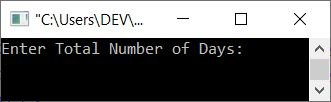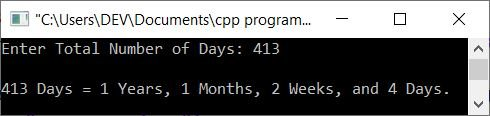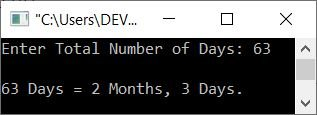# C++ Program to Convert Days into Years, Months, Weeks, and Days

This article provides some programs in C++ that are used to convert the total number of days entered by the user at run-time into years, months, weeks, and days.

For example, if the user enters 406 (the total number of days) as input, the output will be 1 year, 1 month, 1 week, and 4 days. Here is the conversion that shows how 406 days get converted into years, months, weeks, and days:

```406 days = 1 (1*365) + 1 (1*30) + 1 (1*7) + 4
= 1 (year) + 1 (month) + 1 (week) + 4 (days)
= 1 (1*365) + 1 (1*30) + 1 (1*7) + 4
= 365 + 30 + 7 + 4
= 395 + 11
= 406```

The question is, "Write a C++ program that receives the total number of days as input and converts and prints given days into their respective years, months, weeks, and days." The program given below is the answer to this question:

```#include<iostream>

using namespace std;
int main()
{
int tot, days, weeks, months, years;
cout<<"Enter Total Number of Days: ";
cin>>tot;
years = tot/365;
months = (tot%365)/30;
weeks = ((tot%365)%30)/7;
days = ((tot%365)%30)%7;

cout<<"\nYears: "<<years<<endl;
cout<<"Months: "<<months<<endl;
cout<<"Weeks: "<<weeks<<endl;
cout<<"Days: "<<days<<endl;
cout<<endl;
return 0;
}```

The snapshot given below shows the initial output produced by the above C++ program on converting the total number of days into years, months, weeks, and days:Now supply the input, say 406 as the total number of days, and press the ENTER key to see the output as shown in the snapshot given below:When the user enters 406 days into the above program, the tot variable is initialized. Now the statement:

`years = tot/365;`

Because the years variable is of the int type, it returns 1. Therefore, 1 gets initialized to years. And the statement:

`months = (tot%365)/30;`

(tot%365)/30 equals (406%365)/30 equals (41)/30 equals 1. Therefore, 1 gets initialized to months. And the statement:

`((tot%365)%30)/7;`

((tot%365)%30)/7 equals ((406%365)%30)/7 equals ((41)%30)/7 equals (11)/7 equals 1. Therefore, 1 gets initialized to weeks. And the last statement is:

`((tot%365)%30)%7`

((tot%365)%30)%7 equals ((406%365)%30)%7 equals ((41)%30)%7 equals 11%7 equals 4. Therefore, 4 gets initialized to days. In this way, the calculation goes like this, or the evaluation of all four conversion statements goes like this.

Let's replace the above program with the one given below. This program provides a poor user experience because the output gets printed in one line instead of multiples as in the previous program

```#include<iostream>

using namespace std;
int main()
{
int tot, days, weeks, months, years;
cout<<"Enter Total Number of Days: ";
cin>>tot;
years = tot/365;
months = (tot%365)/30;
weeks = ((tot%365)%30)/7;
days = ((tot%365)%30)%7;

cout<<endl<<tot<<" Days = ";
cout<<years<<" Years, ";
cout<<months<<" Months, ";
cout<<weeks<<" Weeks, and ";
cout<<days<<" Days.";
cout<<endl;
return 0;
}```

Here is its sample run with user input of 413 as the number of days:Still, the program appears strange because 1 years must be converted to 1 year (without the s). Let's completely modify the above program:

```#include<iostream>

using namespace std;
int main()
{
int tot, days, weeks, months, years;
cout<<"Enter Total Number of Days: ";
cin>>tot;
years = tot/365;
months = (tot%365)/30;
weeks = ((tot%365)%30)/7;
days = ((tot%365)%30)%7;

cout<<endl<<tot<<" Days = ";
if(years>1)
cout<<years<<" Years, ";
else
{
if(years==1)
cout<<years<<" Year, ";
}
if(months>1)
cout<<months<<" Months, ";
else
{
if(months==1)
cout<<months<<" Month, ";
}
if(weeks>1)
cout<<weeks<<" Weeks, and ";
else
{
if(weeks==1)
cout<<weeks<<" Week, and ";
}
if(days>1)
cout<<days<<" Days.";
else
{
if(days==1)
cout<<days<<" Day.";
}
cout<<endl;
return 0;
}```

Here is its sample run with user input of 63 days:As you can see from the above program's sample run, if any term like year, month, week, or day gets equal to 0, then the output of that term is not shown.

C++ Quiz

« Previous Program Next Program »